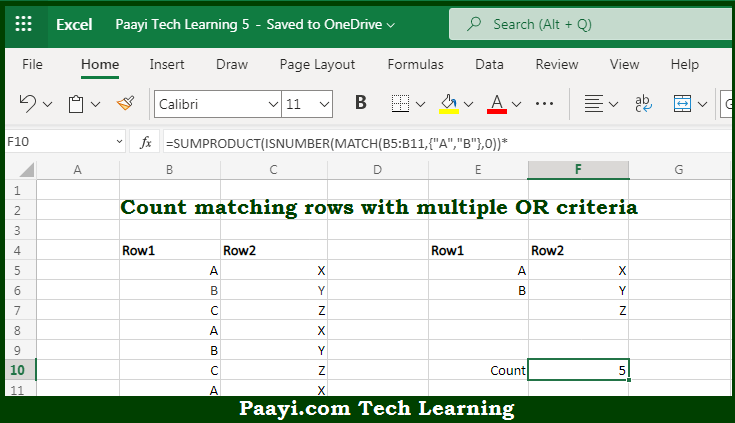# Learn How to SUMPRODUCT Count Multiple OR Criteria in Microsoft Excel

Written by | 0 Comments | 612 Views

In this article, you will learn how to COUNT various things in Microsoft Excel using a single/combination(s) of functions. You will also know how to SUMPRODUCT Count Multiple OR Criteria and see the generic formula.

SUMPRODUCT Count Multiple OR Criteria in Microsoft Excel

The main purpose of this formula is to count matching rows with multiple OR criteria. Here we will learn how to create a summary count with multiple OR criteria in the Microsoft Excel. That implies, with the help of formula based on the SUMPRODUCT function you can able to count matching rows with multiple OR criteria. So, with the help of this formula, you can able to create a summary count with multiple OR criteria in the Microsoft Excel.

General Formula to SUMPRODUCT Count Multiple OR Criteria

=SUMPRODUCT(ISNUMBER(MATCH(rng1,{"A","B"},0))*ISNUMBER(MATCH(rng2,{"X","Y","Z"},0)))

The Explanation for the SUMPRODUCT Count Multiple OR CriteriaSo we know that with the help of the given formula above you can able to generate a count by matching rows with multiple OR criteria. Here we will learn how to create a summary count with multiple OR criteria in Microsoft Excel. As we know that when we work from the inside out, each criterion is will be applicable with a separate ISNUMBER + MATCH construction. So, to generate a count of rows in column one. MATCH generates a result array and ISNUMBER converts this array to another array and To generate a count of rows in column two where the value is given in the F column. So, with the help of this formula, you can able to create a summary count with multiple OR criteria in Microsoft Excel.